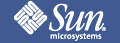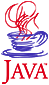JDK Contents

# Math

The math package provides two new classes: `BigInteger` and `BigDecimal`. `BigInteger` numbers are immutable arbitrary-precision integers, which provide analogs to all of Java's primitive integer operators, and all relevant static methods from `java.lang.Math`. Additionally, `BigInteger` numbers provide operations for modular arithmetic, GCD calculation, primality testing, prime generation, single-bit manipulation, and a few other odds and ends.
`BigDecimal` numbers are immutable, arbitrary-precision signed decimal numbers, suitable for monetary calculations. `BigDecimal` numbers provide operations for basic arithmetic, scale manipulation, comparison, format conversion and hashing.

Math API ReferenceCopyright © 1996 Sun Microsystems, Inc., 2550 Garcia Ave., Mtn. View, CA 94043-1100 USA. All rights reserved.